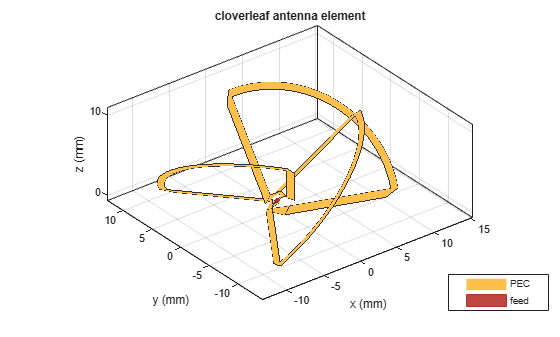# cloverleaf

Create three-petal cloverleaf antenna

## Description

Use the `cloverleaf` object to create a three-petal cloverleaf antenna. The default cloverleaf has 3 petals and operates at around 5.8 GHz. It has a wideband circular polarization and an omnidirectional antenna.## Creation

### Syntax

``cl = cloverleaf``
``cl = cloverleaf(Name,Value)``

### Description

example

````cl = cloverleaf` creates a three-petal cloverleaf antenna. ```
````cl = cloverleaf(Name,Value)` sets properties using one or more name-value pairs. For example, ```cl = cloverleaf('NumPetals',4)``` creates a five-petal cloverleaf antenna. Enclose each property name in quotes.```

## Properties

expand all

Number of petals, specified as a scalar.

Example: `'NumPetals',4`

Example: `cl.NumPetals = 4`

Data Types: `double`

Total length of leaf, specified as a scalar in meters.

Example: `'PetalLength',0.0025`

Example: `cl.PetalLength = 0.0025`

Data Types: `double`

Leaf strip width, specified as a scalar in meters.

Example: `'PetalWidth'`,`0.001`

Example: `cl.PetalWidth = 0.001`

Data Types: `double`

Leaf flare angle, specified as a scalar in degrees.

Example: `'FlareAngle'`,`100`

Example: `cl.FlareAngle = 100`

Data Types: `double`

Type of the metal used as a conductor, specified as a metal material object. You can choose any metal from the `MetalCatalog` or specify a metal of your choice. For more information, see `metal`. For more information on metal conductor meshing, see Meshing.

Example: ```m = metal('Copper'); 'Conductor',m```

Example: ```m = metal('Copper'); ant.Conductor = m```

Lumped elements added to the antenna feed, specified as a lumped element object. You can add a load anywhere on the surface of the antenna. By default, it is at the origin. For more information, see `lumpedElement`.

Example: `'Load',lumpedelement`. `lumpedelement` is the object for the load created using `lumpedElement`.

Example: ```cl.Load = lumpedElement('Impedance',75)```

Data Types: `double`

Tilt angle of the antenna, specified as a scalar or vector with each element unit in degrees. For more information, see Rotate Antennas and Arrays.

Example: `Tilt=90`

Example: `Tilt=[90 90]`,`TiltAxis=[0 1 0;0 1 1]` tilts the antenna at 90 degrees about the two axes defined by the vectors.

Note

The `wireStack` antenna object only accepts the dot method to change its properties.

Data Types: `double`

Tilt axis of the antenna, specified as:

• Three-element vector of Cartesian coordinates in meters. In this case, each coordinate in the vector starts at the origin and lies along the specified points on the X-, Y-, and Z-axes.

• Two points in space, each specified as three-element vectors of Cartesian coordinates. In this case, the antenna rotates around the line joining the two points in space.

• A string input describing simple rotations around one of the principal axes, 'X', 'Y', or 'Z'.

Example: `TiltAxis=[0 1 0]`

Example: `TiltAxis=[0 0 0;0 1 0]`

Example: `TiltAxis = 'Z'`

Note

The `wireStack` antenna object only accepts the dot method to change its properties.

Data Types: `double`

## Object Functions

 `show` Display antenna, array structures or shapes `info` Display information about antenna or array `axialRatio` Axial ratio of antenna `beamwidth` Beamwidth of antenna `charge` Charge distribution on antenna or array surface `current` Current distribution on antenna or array surface `design` Design prototype antenna or arrays for resonance around specified frequency `efficiency` Radiation efficiency of antenna `EHfields` Electric and magnetic fields of antennas; Embedded electric and magnetic fields of antenna element in arrays `impedance` Input impedance of antenna; scan impedance of array `mesh` Mesh properties of metal, dielectric antenna, or array structure `meshconfig` Change mesh mode of antenna structure `optimize` Optimize antenna or array using SADEA optimizer `pattern` Radiation pattern and phase of antenna or array; Embedded pattern of antenna element in array `patternAzimuth` Azimuth pattern of antenna or array `patternElevation` Elevation pattern of antenna or array `rcs` Calculate and plot radar cross section (RCS) of platform, antenna, or array `returnLoss` Return loss of antenna; scan return loss of array `sparameters` Calculate S-parameter for antenna and antenna array objects `vswr` Voltage standing wave ratio of antenna

## Examples

collapse all

Create and view a default cloverleaf antenna.

`cl = cloverleaf`
```cl = cloverleaf with properties: NumPetals: 3 PetalLength: 0.0515 PetalWidth: 8.0000e-04 FlareAngle: 105 Conductor: [1x1 metal] Tilt: 0 TiltAxis: [1 0 0] Load: [1x1 lumpedElement] ```
`show(cl)`Create a cloverleaf antenna.

```cl = cloverleaf; show(cl);```Plot the axial ratio of the antenna from 5 GHz to 6 GHz.

```freq = linspace(5e9,6e9,101); axialRatio(cl,freq,0,0);```The axial ratio plot shows that the antenna supports circular polarization over the entire frequency range.

## Version History

Introduced in R2017b